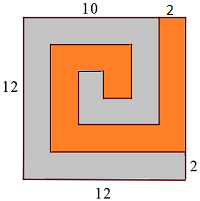# SAT Composite Figures

Geometry Level 2The gray and orange spirals above have a width of 2. What is the area of the gray spiral?

(A) $\ \ 72$
(B) $\ \ 80$
(C) $\ \ 84$
(D) $\ \ 88$
(E) $\ \ 124$

×